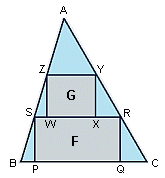All about flooble | fun stuff | Get a free chatterbox | Free JavaScript | Avatarsperplexus dot infoPacking 'em In (Posted on 2004-03-02)ABC is an acute-angled triangle with area 1. A rectangle F(PQRS) has its vertices on the sides of the triangle, with P and Q on BC, R on AC, and S on AB. Another rectangle, G(WXYZ), has its vertices on the sides of triangle ASR, with W and X on RS, Y on AS, and Z on AR.

What is the maximum total area of F and G?

 See The Solution Submitted by DJ Rating: 4.2000 (10 votes)Comments: ( Back to comment list | You must be logged in to post comments.)The Calculus| Comment 6 of 13 |So long as the base and the altitude are the same, the result will be the same regardless of whether the triangle is isosceles or not, so we'll make it isosceles.

The results will be proportional to the height and base of the triangle, so we can assume a standard triangle with altitude 1 and base 1, and scale up the results.

Call the length of an altitude of the triangle AZY x, and the altitude of triangle ASR y.

Rectangle F has height (1-x) and width equal to x.

Rectangle G has height (x-y) and width equal to y.

The total area of the two rectangles is then A = (1-x)x + (x-y)y, which is equal to

A = x - x² + yx - y²

∂A/∂x = 1 - 2x + y = 0

∂A/∂y = x - 2y = 0

so x=2y and 1 - 4y + y = 0, so y = 1/3.

The horizontal lines for the rectangles are 1/3 and 2/3 of the way down the triangle to maximize the area.

Then A = (1/3)(2/3) + (1/3)(1/3) = 1/3 for a triangle whose area is 1/2.

Scaling up, A = base * height / 3, or 2/3 the area of the triangle as a whole.

 Posted by Charlie on 2004-03-02 15:36:59Please log in:
 Login: Password: Remember me: Sign up! | Forgot password

 Search: Search body:
Forums (0)
Newest Problems
Random Problem
FAQ | About This Site
Site Statistics
New Comments (0)
Unsolved Problems
Top Rated Problems
This month's top
Most Commented On

Chatterbox:
Copyright © 2002 - 2021 by Animus Pactum Consulting. All rights reserved. Privacy Information